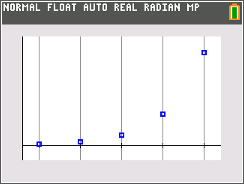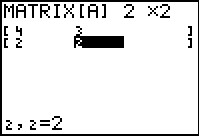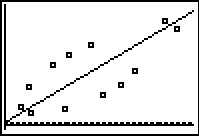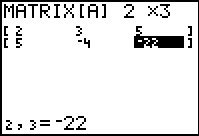Outbreak!

Students explore a geometric sequence related to an outbreak of the flu.
•TI-84 Plus CE
•TI-84 Plus C Silver Edition
• TI-84 Plus Silver Edition
• TI-84 PlusExploring Geometric Sequences

Students graphically analyze geometric series using graphs and consider the effect of the value for the common ratio and first term using sliders.
•TI-84 Plus CE
•TI-84 Plus C Silver Edition
• TI-84 Plus Silver Edition
• TI-84 PlusHow Many? (Precalculus)

Students will be presented a situation in which they must use linear programming to determine the optimum production level to maximize profits.
•TI-84 Plus CE
•TI-84 Plus C Silver Edition
• TI-84 Plus Silver Edition
• TI-84 PlusAll Systems Go!

Students learn about inverse matrices in the context of encoding/decoding secret messages and then use inverse matrices to solve systems of equations.
•TI-84 Plus CE
•TI-84 Plus C Silver Edition
• TI-84 Plus Silver Edition
• TI-84 PlusInfinite Geometric Series

Students explore infinite geometric series. They will consider the effect of the value for the common ratio and determine whether an infinite geometric series converges or diverges.
• TI-84 Plus Silver Edition
• TI-84 PlusInverses of Functions

Students explore three ways to find the inverse of a function. First, students graph two scatter plots and find the line of reflection. Then, they will graph a line and use the x- and y-intercepts to create the graph of the inverse.
•TI-84 Plus CE
•TI-84 Plus C Silver Edition
• TI-84 Plus Silver Edition
• TI-84 Plus••